# Some computational redistricting methods

The last in a series of notes on gerrymandering & R. Here, an example application of some of the computational redistricting methods presented in ; ; ; ; , and an implementation of redist, a redistricting simulation package in R. || For demonstration purposes: legislative boundaries (2012) and election results (2016) for the New Mexico State Senate. Importantly, code presented here is super-flexible, and will easily scale to different states and different legislative bodies.

Basic gist: investigate the representativeness of a proposed redistricting plan by comparing (1) the vote outcome based on proposed plan, and (2) vote outcomes based on an ensemble of randomly partitioned redistricting plans – where a “vote outcome” is a simulated election result based on precinct-level returns from some historical election.

## Statewide historical election data

The MGGG States project provides an open collection of precinct shapefiles for US states. Here, we consider the state of New Mexico; the shapefile for which includes precinct-level results for six statewide elections.

library(tidyverse)
nm_sf <- sf::st_read(dsn = paste0(gitdir, # local folder
fname), # shapefile name from MGGG --
layer = fname,
quiet = TRUE) %>%

## as simple df for viewing --
nm_df <- nm_sf %>%
data.table::data.table() %>%
select(-geometry) 

Column names below. So, lots of information compiled/included in the shapefile; in addition to district details and election returns, precinct-level race and ethnicity counts are also made available.

colnames(nm_sf)
##   "NAME10"    "prec_num"  "County"    "AG18D"     "AG18R"     "AG18L"
##   "SOS18D"    "SOS18L"    "SEN18R"    "SEN18D"    "SEN18L"    "PRES16L"
##  "PRES16R"   "PRES16D"   "SOS16R"    "SOS16D"    "GOV18D"    "GOV18R"
##  "CDDIST"    "HDIST"     "SDIST"     "TOTPOP"    "NH_WHITE"  "NH_BLACK"
##  "NH_AMIN"   "NH_ASIAN"  "NH_NHPI"   "NH_OTHER"  "NH_2MORE"  "HISP"
##  "H_WHITE"   "H_BLACK"   "H_AMIN"    "H_ASIAN"   "H_NHPI"    "H_OTHER"
##  "H_2MORE"   "VAP"       "HVAP"      "WVAP"      "BVAP"      "AMINVAP"
##  "ASIANVAP"  "NHPIVAP"   "OTHERVAP"  "X2MOREVAP" "Area"      "Perimeter"
##  "SOS18R"    "geometry"

In the following analyses, we focus on voting results from four historical statewide elections:

geos <- c('NAME10', 'prec_num', 'County', 'SDIST')

short <- c('SEN18', 'GOV18', 'PRES16', 'SOS16')

election <- c('2018 Senate',
'2018 Governor',
'2016 President',
'2016 Secretary of State')
short election
SEN18 2018 Senate
GOV18 2018 Governor
PRES16 2016 President
SOS16 2016 Secretary of State

Lastly, some data re-structuring. The resulting returns data frame contains precinct-level vote returns disaggregated by party and election, in long format.

ecs <- grep(paste0(esum$short, collapse = '|'), colnames(nm_df), value = T) returns <- data.table::melt(nm_df, geos, ecs) %>% mutate(party = gsub('^.*[0-9]', '', variable), election = gsub('.$', '', variable)) %>%
select(-variable)

## New Mexico State Senate

The existing legislative map for the New Mexico State Senate was drawn during the 2010 cycle and, after a hiccup or two, went into effect in time for the general election in 2012. This partition will be referred to as NM2012.

#NAME10, partition, district
partition2012 <- nm_df %>%
mutate(partition = 'NM2012') %>%
mutate(district = as.character(as.numeric(SDIST))) %>%
select(NAME10, partition, district)

Election results from 2016 are available via the nmelectiondatr package – an election in which all 42 seats were up for election. Dems won 26/42 seats. Note that Senate elections were most recently held in 2020; however, for our purposes here, 2016 is fine.

nm16 <- nmelectiondatr::nmel_results_summary %>%
filter(Type == 'State Senator',
Year == '2016') %>%
rename(district = Type_Sub,
party = Party) %>%
mutate(party = substr(party, 1,1),
partition = 'NM2016')

## Building an ensemble

The redist package includes multiple approaches to randomly sampling graph partitions; here, we implement the Sequential Monte Carlo (SMC) method via the redist.smc function. The SMC method requires two intermediary spatial data structures to build our ensemble of redistricting plans: (1) a distance matrix, which is derived from centroids of the precinct-level shapefile, and (2) an adjacency matrix.

centers <- sf::st_centroid(nm_sf) # %>% select(NAME10))
distmat <- sf::st_distance(centers)
attr(distmat, "units") <- NULL
distmat <- sqrt(distmat)

adj_list <- redist::redist.adjacency(nm_sf)

Parameters of relevance: (1) popvec: a vector specifying precinct-level voting age population counts; (2) ndists: the number of legislative boundaries to create; (3) nsim: the number of partitions to create; (4) popcons: the weight of population constraint; and (5) compactness: the weight of the compactness constraint.

Building the ensemble, then, amounts to piecing together 42 districts from 1,483 precincts – 10,000 times over – while abiding by the population & compactness constraints.

set.seed(9999)
popvec = nm_sf$VAP, nsims = 10000, ndists = 42, popcons = 0.2, compactness = 1) ## Sampling 10000 1483-unit maps with 42 districts and population tolerance 20%. The ensemble of 10K partitions is included in model output as a matrix. Partitions are not shapefiles or geometries; instead, each partition is represented as a vector in which each precinct is assigned a district number. ensembles <- smc$cdvec %>%
data.table::data.table() %>%
mutate(NAME10 = nm_df$NAME10) %>% data.table::melt(., 'NAME10', c(1:ncol(smc$cdvec))) %>%
rename(partition = variable,
district = value) %>%
mutate(partition = as.character(partition),
district = as.character(district)) %>%
bind_rows(partition2012) ###

## sample --
set.seed(99)
samp4 <- c('NM2012',
sample(unique(ensembles$partition), size = 3)) Sample of partition matrix re-structured as a data frame: NAME10 partition district Catron County Precinct 6 V1 42 Catron County Precinct 3 V1 42 Catron County Precinct 4 V1 42 Catron County Precinct 2 V1 38 Catron County Precinct 1 V1 42 Catron County Precinct 5 V1 38 lc <- tmaptools::geocode_OSM (q = 'Albuquerque, NM', as.sf = T) lc$bbox <- sf::st_set_crs(lc$bbox, sf::st_crs(nm_sf)) cropped <- sf::st_crop(nm_sf, lc$bbox)

The map below illustrates an example partition, zoomed into the Albuquerque Metro Area for a better look at things. Color reflects precinct-level district assignment per the SMC algorithm.

dists <- 42
nc <- cropped %>%
select(-prec_num:-SOS18R) %>%
left_join(ensembles %>% filter(partition %in% samp4),
by = 'NAME10')

nc %>%
filter(partition == samp4[]) %>%
ggplot() +
geom_sf(aes(fill = district),
color = 'white',
alpha = .65,
lwd = .1) +
scale_fill_manual(
values = colorRampPalette(ggthemes::stata_pal()(8))(dists)) +
theme_minimal() + map_theme() +
labs(title = paste0('Partition ', samp4[]),
subtitle = 'ABQ Metro Area')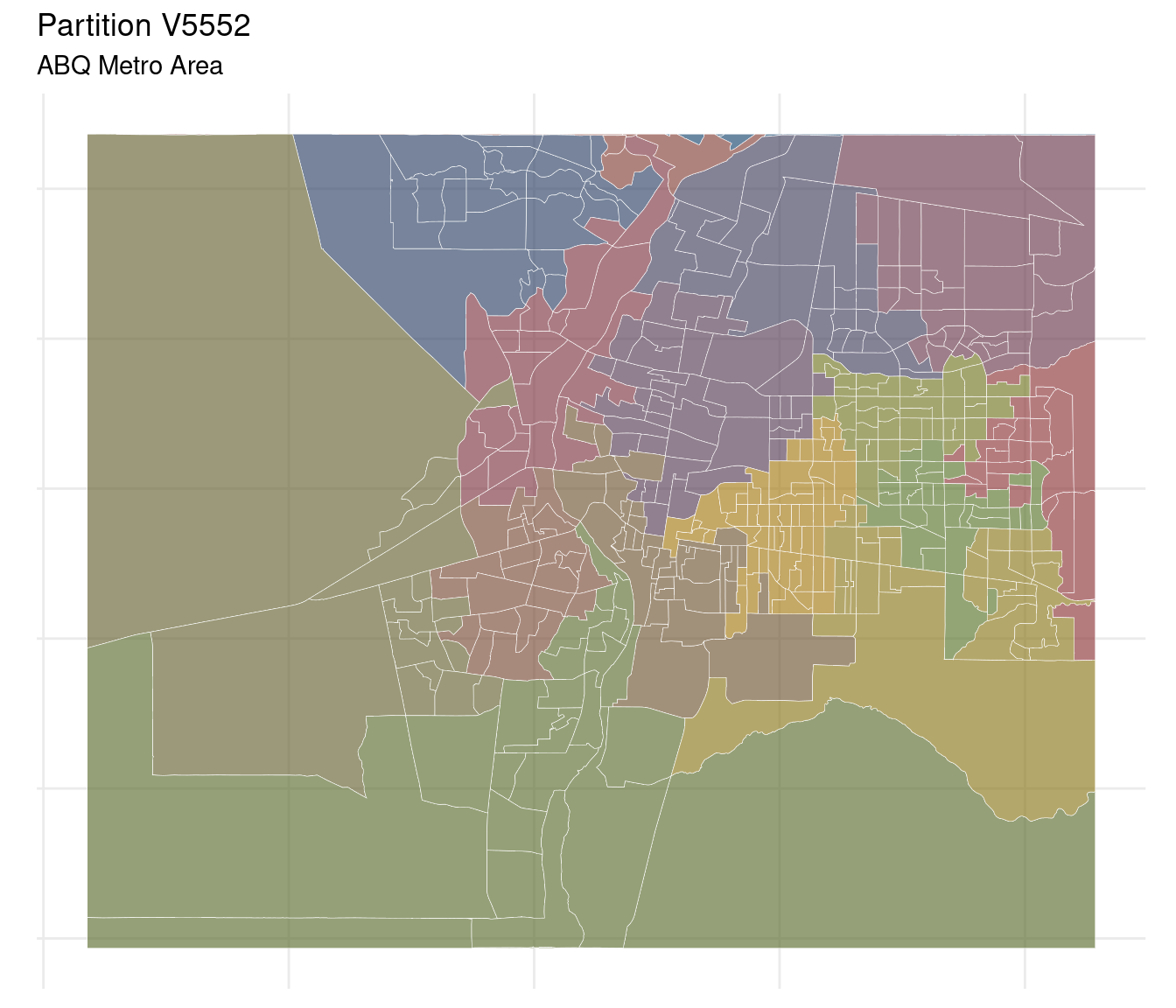We then aggregate precincts by district assignment to build out formal legislative boundaries for partition V5552.

nc %>%
filter(partition == samp4[]) %>%
group_by(partition, district) %>%
summarise(geometry = sf::st_union(geometry)) %>%
ungroup() %>%

ggplot() +
geom_sf(aes(fill = district),
color = 'white',
alpha = .65,
lwd = .3) +
geom_sf_text(aes(label = district),
color = 'black',
size = 2.5,
check_overlap = TRUE) +
scale_fill_manual(
values = colorRampPalette(ggthemes::stata_pal()(8))(dists)) +
theme_minimal() + map_theme() +
labs(title = paste0('Partition ', samp4[]),
subtitle = 'ABQ Metro Area')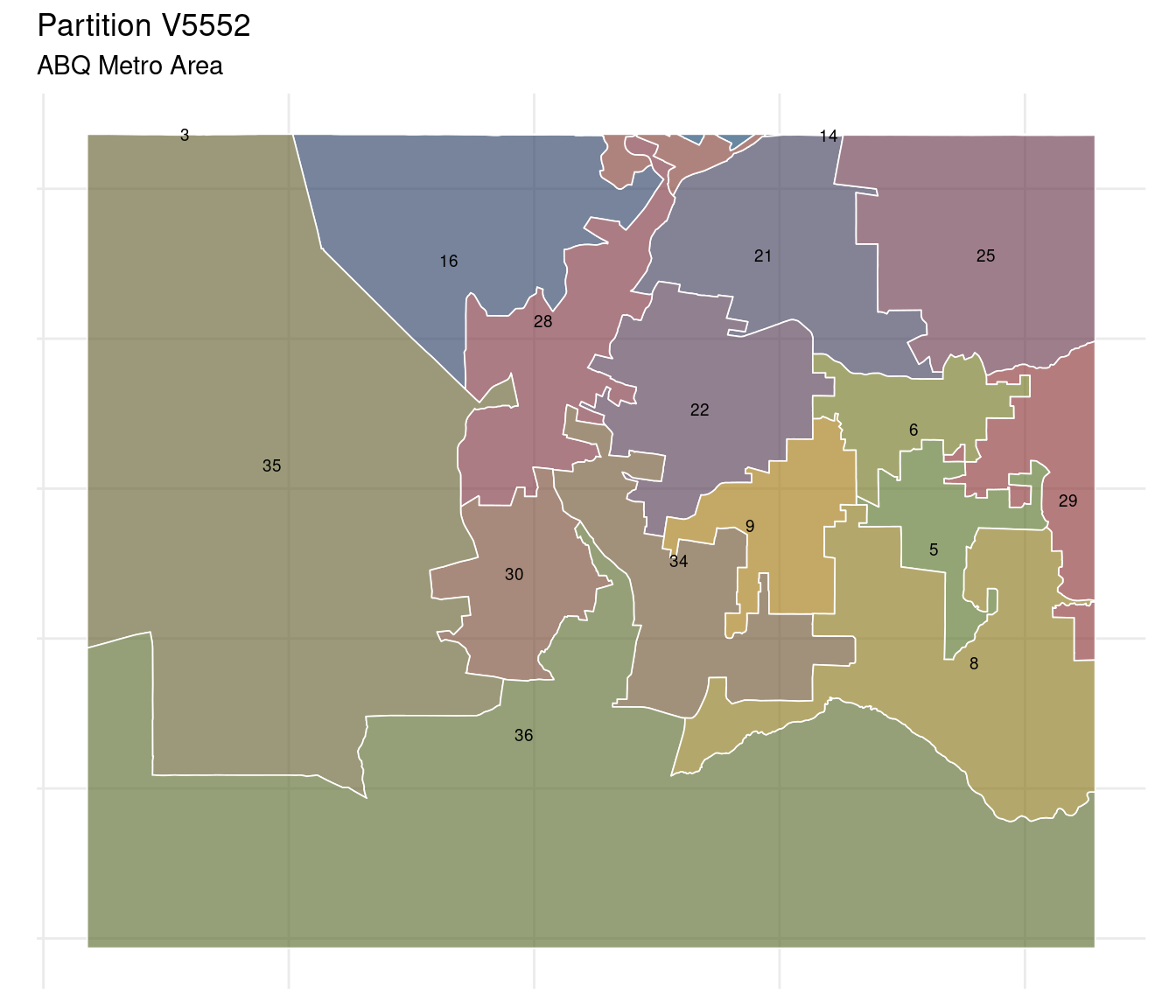## Partition ensemble & historical votes

returns1 <- returns %>%
select(NAME10, election, party, value) %>%
filter(party %in% c('R', 'D'))

From redistricting plan to election result, then, is fairly straightforward. The first step is to assign each partition in our ensemble voting data – again, using election results from four historical elections in New Mexico.

ps <- unique(ensembles$partition) fens <- list() for (q in 1:length(ps)) { x <- subset(ensembles, partition == ps[q]) x1 <- x[returns1, on = 'NAME10'] fens[[q]] <- x1[ , list(value = sum(value)), by = list(election, partition, district, party)] print(q) } x2 <- fens %>% data.table::rbindlist() As a simple starting point, the map below details precinct-level election results in the ABQ Metro Area for the 2018 statewide race for Governor. The darker the shade of blue, the larger the vote margin in favor of the Democratic candidate (here MLG). c1 <- cropped %>% mutate(g18 = GOV18R - GOV18D) c1 %>% ggplot() + geom_sf(aes(fill = g18), color = 'black', alpha = .85, lwd = .05) + scale_fill_distiller(palette = "RdYlBu", limit = max(abs(c1$g18)) * c(-1, 1)) +
theme_minimal() + map_theme() +
labs(title = '2018 Gubernatorial election results',
subtitle = 'ABQ Metro Area')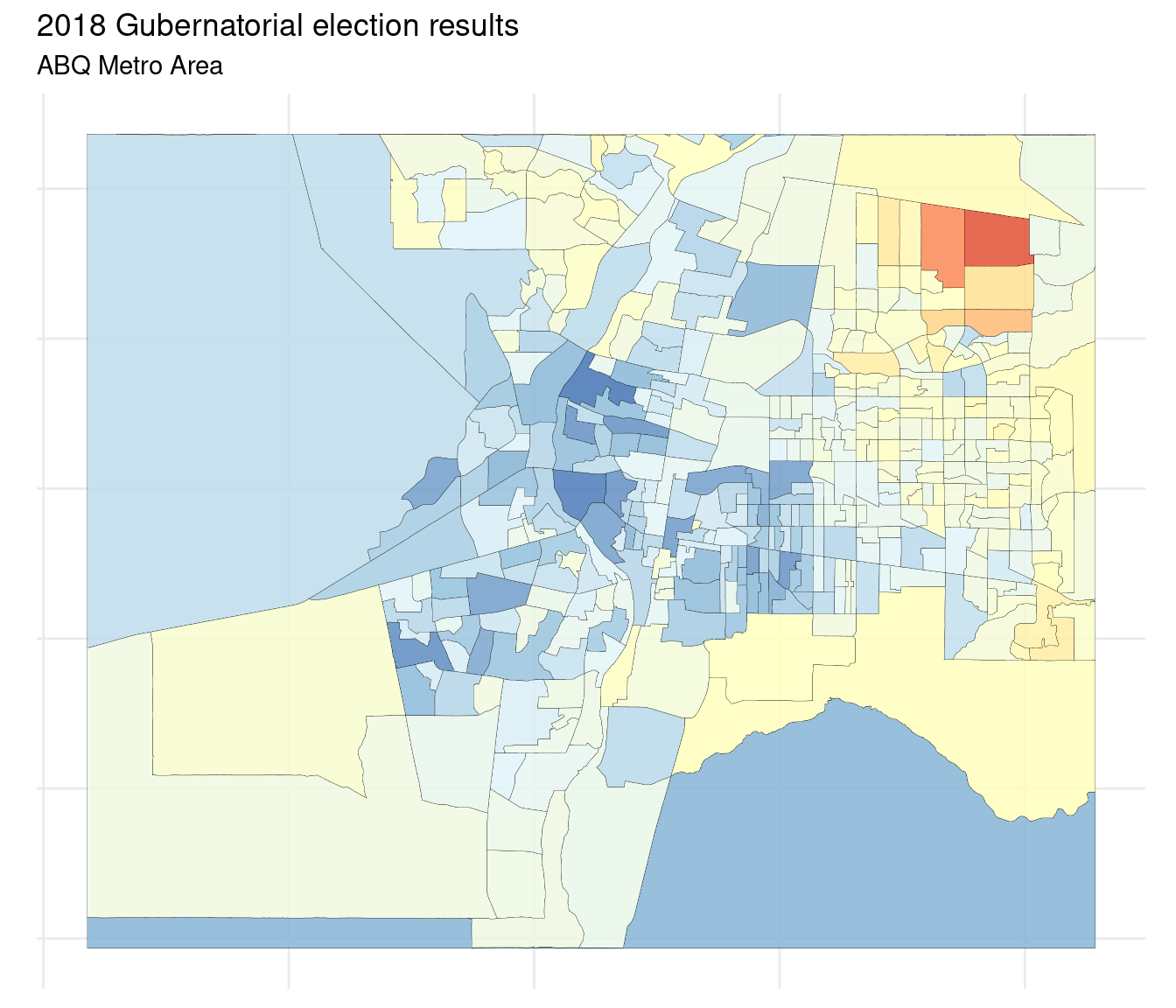nc1 <- nc %>%
group_by(partition, district) %>%
summarise(geometry = sf::st_union(geometry)) %>%
ungroup() 

Then we overlay four partitions on top of these results, three from our randomly generated ensemble, along with the actual NM2012 partition.

ggplot(data = c1) +
geom_sf(aes(fill = g18),
#color = 'black',
alpha = .85,
lwd = .05) +
scale_fill_distiller(palette = "RdYlBu",
limit = max(abs(c1$g18)) * c(-1, 1)) + geom_sf(data = nc1, fill = NA, color = 'black', lwd = .25) + facet_wrap(~partition) + theme_minimal() + map_theme() + labs(title = 'Redistricting plans as overlay', subtitle = 'ABQ Metro Area')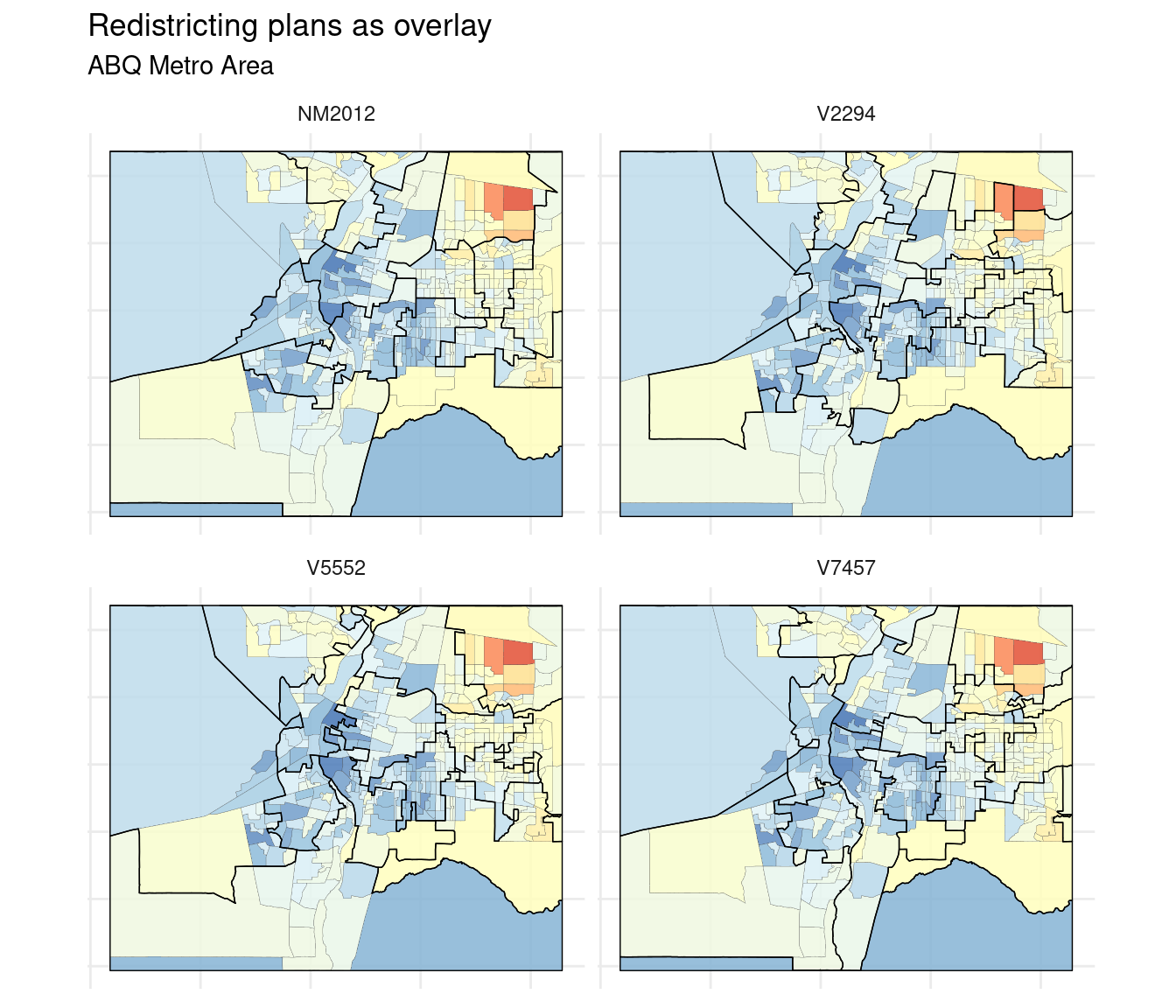winners <- x2 %>% filter(election == 'GOV18') %>% select(-election) %>% filter(partition %in% samp4) %>% ## append Actual partition -- bind_rows(nm16 %>% select(partition, district, party, value)) %>% group_by(partition, district) %>% filter(value == max(value))%>% ungroup()  Lastly, we tabulate election results for our example redistricting plans by aggregating precinct-level voting outcomes from the GOV18 election. The basic gist, then, is to (1) fix precinct-level vote counts, (2) adjust partition, and (3) tabulate results. nc1 %>% left_join(winners) %>% ggplot() + geom_sf(aes(fill = party), color = 'white', alpha = .85, lwd = .25) + geom_sf_text(aes(label = district), color = 'black', size = 2.5, check_overlap = TRUE) + scale_fill_manual(values = c('#678fc3', '#e76a53')) + theme_minimal() + map_theme() + facet_wrap(~partition) + labs(title = 'Redistricting plans: party winners', subtitle = 'ABQ Metro Area')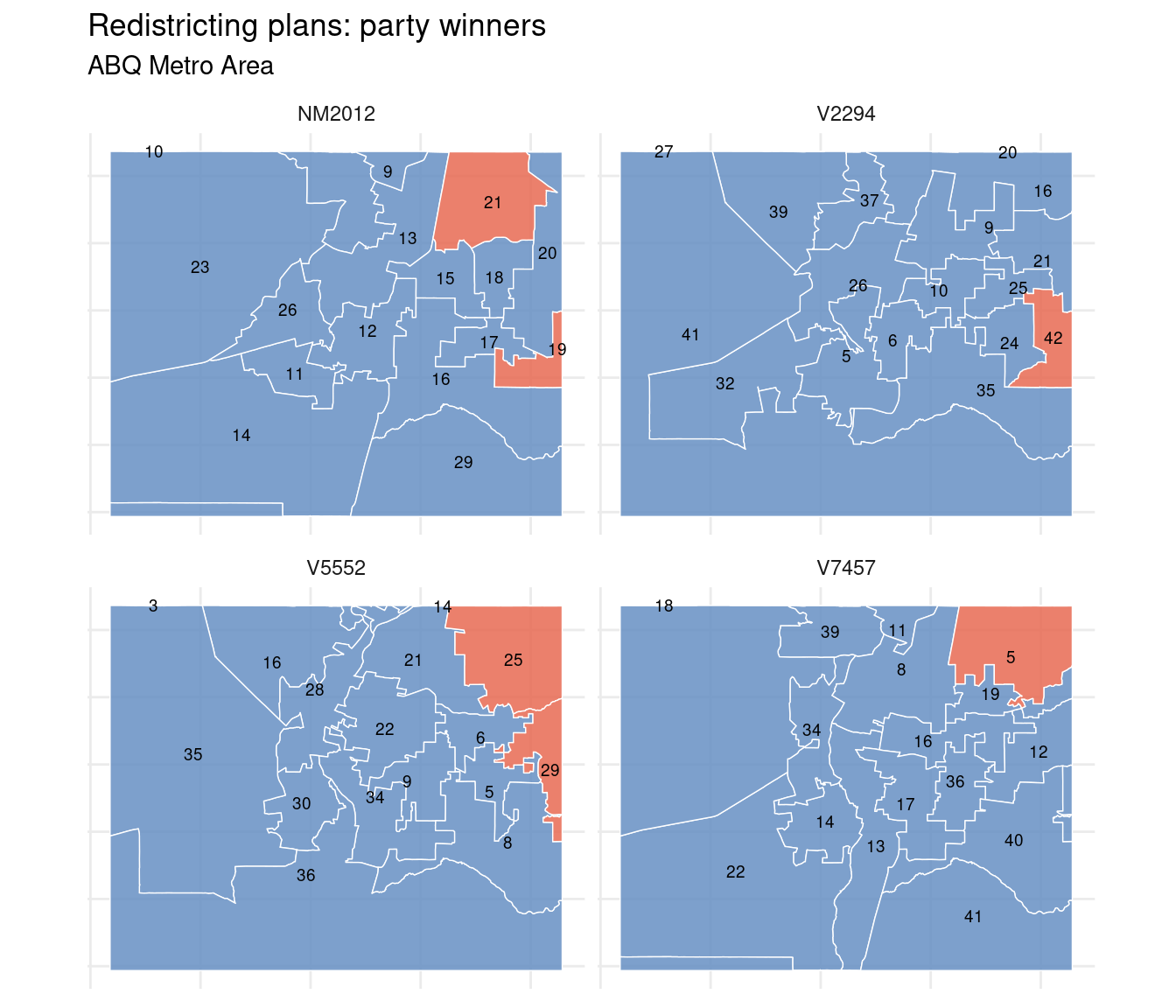## Results So, 10K redistricting plans, four historical elections. In theory, if an existing plan – or some proposed plan – is on the level, it should result in a legislature similar in partisan composition to those generated by the SMC algorithm. comp <- x2 %>% group_by(election, partition, district) %>% filter(party == party[which.max(value)]) %>% group_by(election, partition, party) %>% summarize(seats = n()) %>% ungroup() ### Seat distributions Recall that Dems won 26/42 New Mexico Senate seats in 2016; 27/42 in 2020. ds <- comp %>% filter(party == 'D') ds1 <- ds %>% group_by(election, seats) %>% count() %>% group_by(election) %>% mutate(pertot = round(n/sum(n)*100, 2)) The distribution of the number of elected Democrats using vote outcomes from PRES16 is summarized in the table below. Per these outcomes, ~57% of the 10K redistricting plans resulted in a legislature comprised of more than 27 Dems. election seats n pertot PRES16 23 2 0.02 PRES16 24 39 0.39 PRES16 25 879 8.79 PRES16 26 1392 13.92 PRES16 27 1991 19.91 PRES16 28 4102 41.02 PRES16 29 1436 14.36 PRES16 30 155 1.55 PRES16 31 5 0.05 The faceted plot below details distributions of the number of Democratic wins using vote counts from four historical elections. For context, the number of Democrats actually elected to office in 2016 (26) is highlighted in orange. ds %>% ggplot() + geom_histogram(aes(seats, fill = election), binwidth = .5) + geom_vline(xintercept = 26, linetype = 3, color = '#e37e00', size = 1) + scale_x_continuous(breaks=seq(min(ds$seats), max(ds\$seats),1)) +
facet_wrap(~election) + #, scales = 'free') +
ggthemes::scale_fill_economist()+
theme_minimal()+
theme(legend.position = 'none') +
xlab('Number of Democrats Elected') +
ggtitle('Distributions of elected Democrats')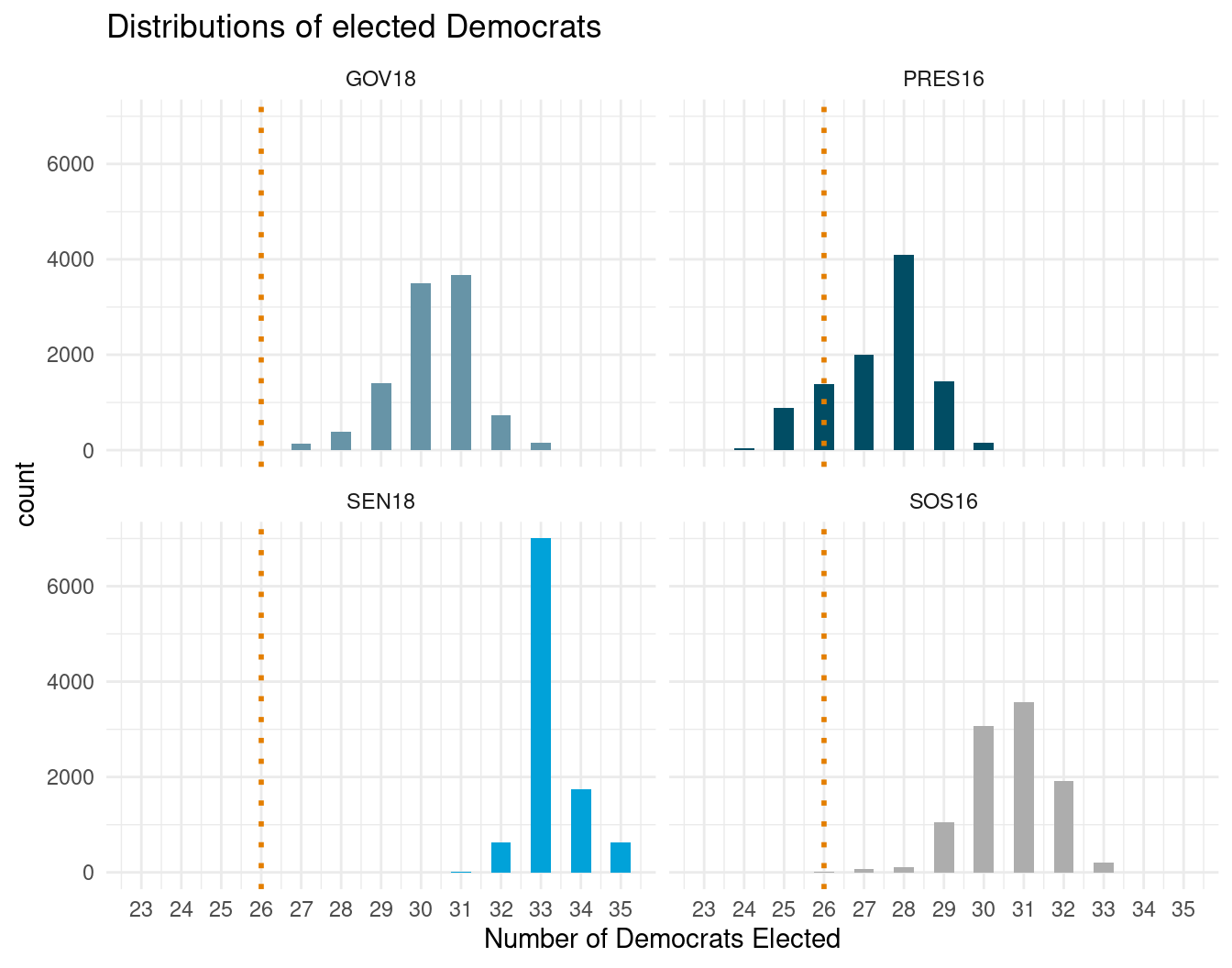Interesting results for sure. The 26-seat Dem outcome only really occurs when using PRES16 election results; while a handful of partitions result in 26 Dem seats for GOV18 and SOS16, over 99% of all plans outside of PRES16 result in legislatures comprised of more than 26 Democrats. For GOV18 and SOS16, elections with very similar result-profiles, the plurality of redistricting plans result in 31 Dem seats. For SEN18, the overwhelming majority of redistricting plans results in 33/42 seats for Dems.

No real explanations here – other than (1) candidates matter, (2) New Mexico’s politics dash leftward, (3) PRES16 was a weird election in NM, as hometown third-party pol Gary Johnson won ~10% statewide, and (4) folks vote differently in statewide-federal elections than in state rep elections. All that said, results from our generated redistricting plans suggest Dems are due a seat or two.

### Marginal distributions

Another way to contextualize a given redistricting plan in relation to our cache of generated plans is by investigating the structure of ordered marginal vote fractions (see, eg, Herschlag et al. 2020).

x3 <- x2 %>%
group_by(election, partition, district) %>%
mutate(per = round(value/sum(value)*100, 3)) %>%
#ungroup() %>%
select(-value) %>%
group_by(election, partition) %>%
mutate(rank = rank(D)) %>%
ungroup()

We have discussed some in previous posts the convention of summarizing election results for a given legislature as a vote distribution; in which Democratic vote shares are plot in ranked order from least to most Democratic. The marginal distribution, then, is the ranked order of vote fractions over the entire ensemble of partitions. The idea being that if a given plan is on the level, its vote distribution should look similar to the marginal distribution of the entire ensemble.

vote_dist <- nm16 %>%
filter(Winner == 'Winner')  %>%
select(district, party, Percent) %>%
mutate(D = ifelse(party == 'R', 1 - Percent, Percent),
# D = ifelse(D == 1, 0.75, D),
# D = ifelse(D == 0, 0.25, D),
rank = rank(D, ties.method = 'first'))

Here, we compare 2016 NM State Senate election results (based on the NM2012 partition) to those of the full ensemble. For each of our four statewide elections, comparisons are illustrated below. Lots of uncontested races in NM Senate 2016 make this particular set of comparisons less than fantastic.

We are basically on the lookout for potential gerrymanders, here in the form of cracking & packing. In theory, rank voting distribution plots should increase ~linearly. Deviations from a linear slope hint at a suspect plan.

While there is nothing definitive along these lines in the plots below, there is a bit of a “wiggle” in the marginal distribution (of our randomly partitioned ensemble) at the transition of Republican-held seats to Democratic-held seats, which suggests a natural gerry/packing of Republicans in (likely) more rural districts in the state.

x3 %>%
ggplot() +
geom_boxplot(aes(factor(rank),
D,
color = election),
size = 0.5,
outlier.size = 0.2) +

geom_point(data = vote_dist,
aes(x = factor(rank),
y = D * 100),
color = '#e37e00',
size = 1) +

ggthemes::scale_color_economist() +
geom_hline(yintercept = 50, lty = 2, color = 'steelblue') +
facet_wrap(~election, ncol = 2) +
theme_minimal() +
theme(axis.text.x=element_blank(),
legend.position = 'none') +
xlab('Districts ordered from least to most Democratic') +
ylab('Percentage of votes for a Democrat') +

ggtitle('Marginal distributions')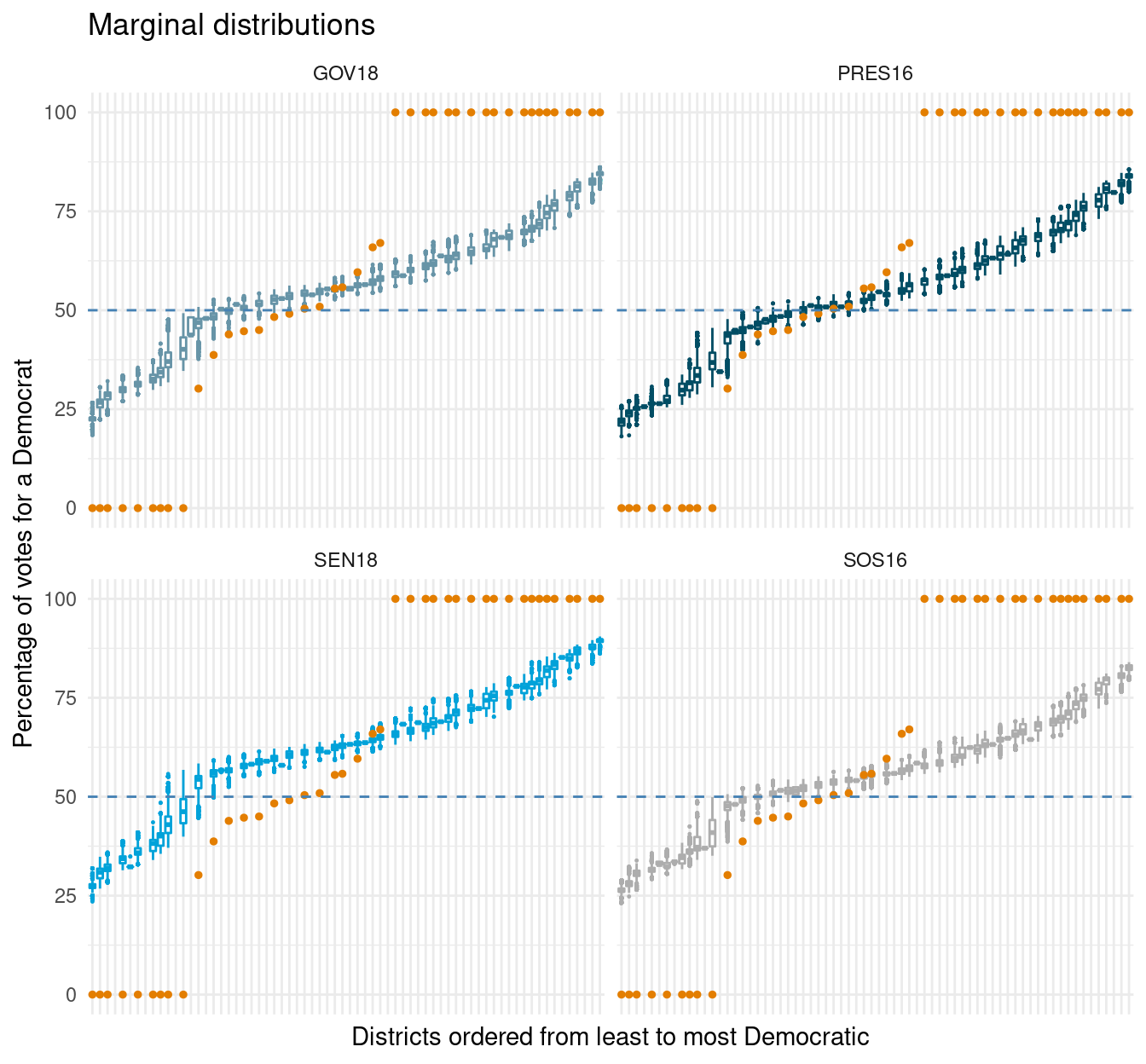### Ranked-marginal deviation

Lastly, we consider a partisan metric introduced in dubbed ranked-marginal deviation. A simple metric, and a natural extension from the marginal distribution plots above, ranked-marginal deviation measures the distance between a given plan and the ordered marginal medians. Any plan, then, can be compared to the ensemble and assigned a similarity score – of sorts. The higher the ranked-marginal deviation, the more likely the plan is suspect.

In-line with the findings from the seat distribution results, the existing NM2012 seems a bit unrepresentative of the state’s politics 10 years on, and likely biased some against Democrats, despite the fact that Dems already hold a sizable 27-15 majority. With caveats galore.

x4 <- x3 %>%
group_by(election, rank) %>%

mutate(med = median(D),
diff = (D - med) ^ 2) %>%
group_by(election, partition) %>%

summarize(marg_dev = sqrt(sum(diff)))

x5 <- x4 %>% filter(partition %in% c(samp4))

x4 %>%
ggplot() +
geom_density(aes(marg_dev, fill = election),
alpha = .5, color = 'gray') +
geom_vline(data = x5,
aes(xintercept = marg_dev),
color = '#e37e00',
linetype = 2,
size = .5) +

geom_text(data = x5,
aes(x = marg_dev, y = 0.3, label = partition),
color = '#e37e00', size = 3,
check_overlap = TRUE) +

theme_minimal() +
theme(legend.position = 'none')+
ggthemes::scale_fill_economist() +
facet_wrap(~election) +
ggtitle('Ranked-Marginal Deviation')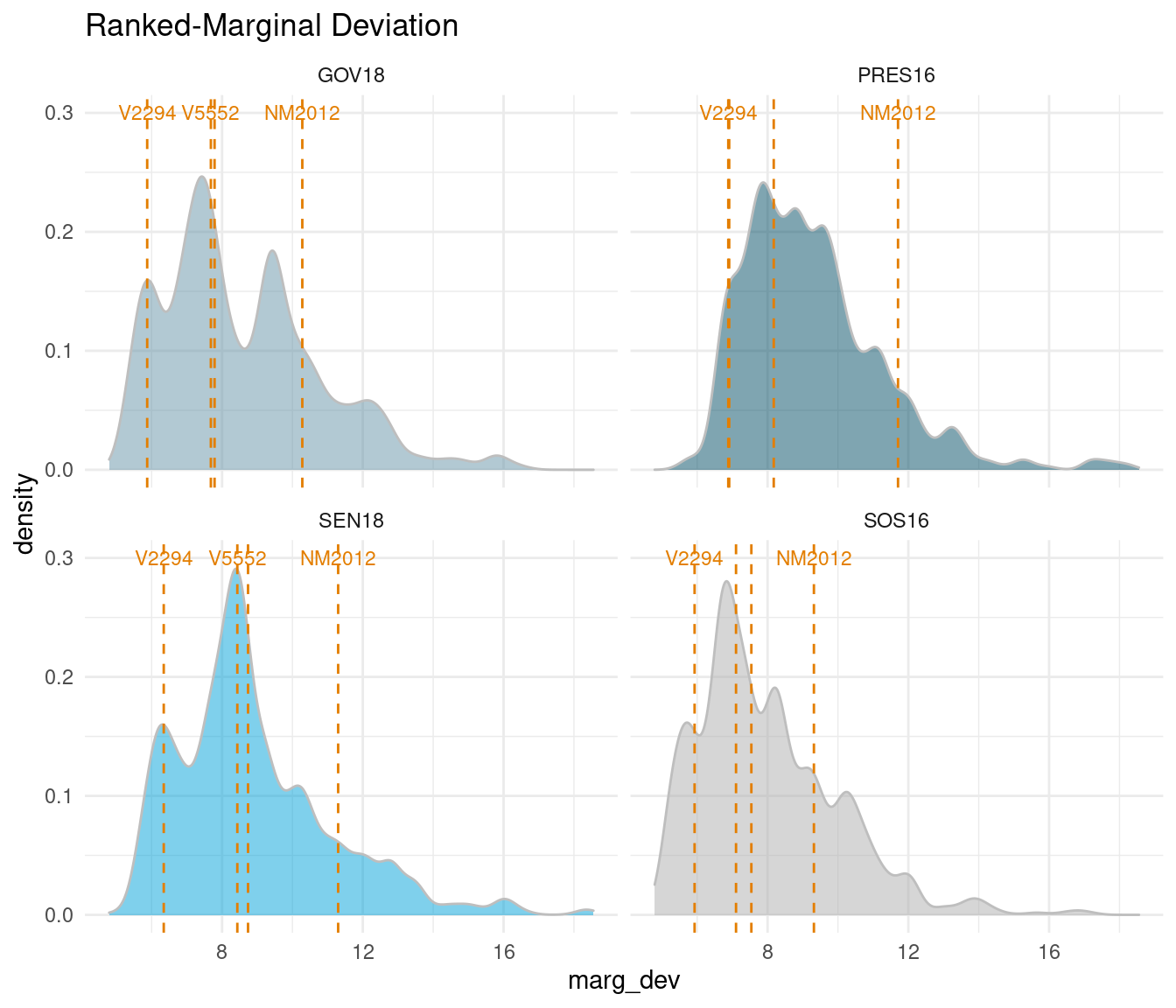## Summary

So, hopefully a useful resource, with the decennial census complete and redistricting in progress. The redist package is a beast of a tool for fair representation – and we only touch the surface of its functionality here. These are basically my learning notes – check out the references for a deeper & proper delve into some fantastic research!!